﻿ The Hydrodynamic Representation of the Klein-Gordon Equation with Self- Interacting Field
Journal of Astrophysics & Aerospace Technology
All submissions of the EM system will be redirected to Online Manuscript Submission System. Authors are requested to submit articles directly to Online Manuscript Submission System of respective journal.
• Research Article
• J Astrophys Aerospace Technol 2017, Vol 5(2): 148
• DOI: 10.4172/2329-6542.1000148

# The Hydrodynamic Representation of the Klein-Gordon Equation with Self- Interacting Field

Piero Chiarelli*
National Council of Research of Italy, Interdepartmental Center “E. Piaggio”, University of Pisa, Area of Pisa, Pisa, Moruzzi 1, Italy
*Corresponding Author: Piero Chiarelli, National Council of Research of Italy, Interdepartmental Center “E. Piaggio”, University of Pisa, Italy, Tel: +39-050-315- 2359, Fax: +39-050-315-2166 , Email: [email protected]

Received Date: Jul 31, 2017 / Accepted Date: Aug 09, 2017 / Published Date: Aug 14, 2017

### Abstract

In this paper the quantum hydrodynamic approach for the KGE owing a self-interaction term is developed both for scalar and charged boson. The model allows to determine the quantum energy impulse tensor density of massive bosons such as the mesons. The generalization of the hydrodynamic Klein-Gordon equation to the non-Euclidean space-time is also derived for a quantum relativity approach.

Keywords: Quantum hydrodynamic representation; Bhom-Madelung approach; Self-interacting field; Non-Euclidean quantumhydrodynamics

#### Introduction

Since the introduction of the quantum wave equation by Schrödinger, the quantum hydrodynamic approach (QHA) was presented by Madelung . In this quantum representation, developed by Madelung and then by Bhom, the evolution of a complex variable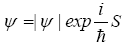is solved as a function of the two real variables,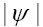and S [2-5]. As shown by Weiner et al. , the outputs of the quantum hydrodynamic model agree with the outputs of the Schrödinger problem and, more recently, as shown by Koide and Kodama , it agrees with the outputs of the stochastic variational method.

Recently, the author has shown that the hydrodynamic approach is strictly correlated to the properties of vacuum on small scale .

Moreover, as shown by Bohm and Hiley [9,10] the hydrodynamic approach can be generalized for the description of the quantum fields.

The present work develops the quantum hydrodynamic form of the Klein- Gordon equation (KGE) containing an additional self-interaction term.

The interest in obtaining such a description lies in the fact that such type of KGE can describe the states of bosons, such as mesons. The goal of the paper is to obtain the energy-impulse tensor density of such particles that can be useful in the coupling the field of a meson with the Einstein equation . The paper is organized as follows: in the section 2 the hydrodynamic KGE with a self-interaction term is derived for an uncharged scalar particle as well as the Lagrangean motion equations for the eigenstates and the associated energy impulse tensor density.. In the subsection 2.2 the theory is developed for a charged field. In section 3. the formulas are generalized to a non-Euclidean space-time.

#### The Hydrodynamic KGE with Self-Interacting Field

In this section, the Euclidean hydrodynamic representation of the KGE is derived for a scalar uncharged particle with a self-interaction term that reads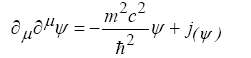(1)

where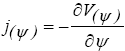and where, for instance, we assume the quartic renormalizable interaction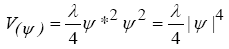Following the procedure given in reference [11,12] (for the ordinary KGE) the hydrodynamic equations of motion are given by the Hamilton-Jacobi type equation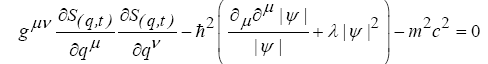(6)

coupled to the current equation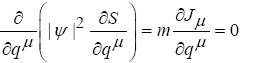(3)

where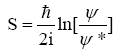(4)

and where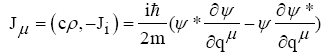(5)

Moreover, being the 4-impulse in the hydrodynamic analogy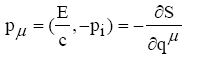(6)

it follows that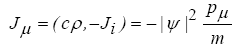(7)

where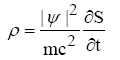(8)

Moreover, by using (6), equation (2) can be rewritten as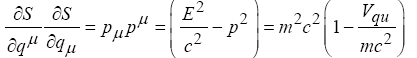(9)

where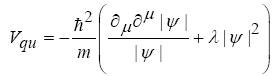(10)

and where P2 = Pi Pi is the modulus of the spatial momentum.

As shown in reference , given the hydrodynamic Lagrangean function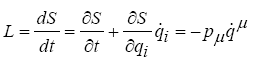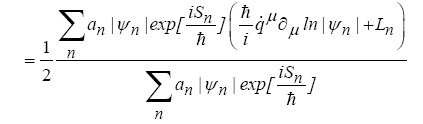(11)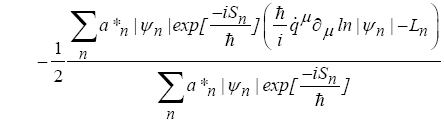equation (2) can be expressed by the following system of Lagrangean equations of motion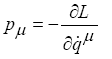(12)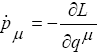(13)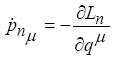(14)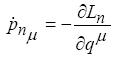(15)

where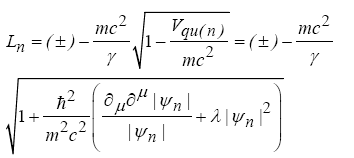(16)

Generally speaking, for eigenstates, for which it holds E=En=const it follows that: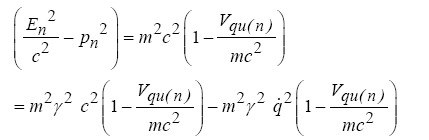(17)

from where it follows that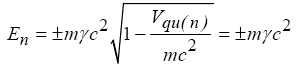(18)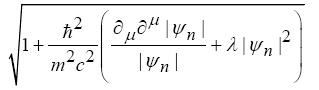(where the minus sign stands for antiparticles) and, by using (17), that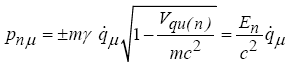(19)

Following the hydrodynamic protocol , the eigenstates are represented by the stationary solutions of the hydrodynamic equations of motion obtained by deriving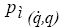from (14) and then inserting it into (15) that leads to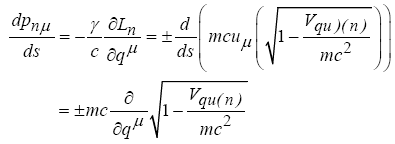(20)

where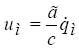and to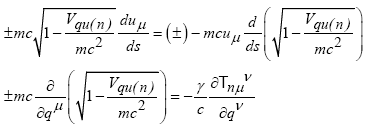(21)

where, for eigenstates, the quantum energy-impulse tensor (QEIT)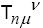reads [11,12],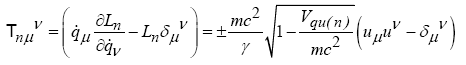(22)

leading to the quantum energy impulse tensor density (QIETD) [11,12],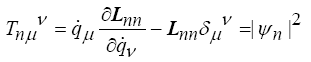(23)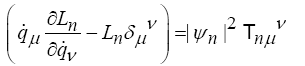where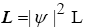is the (hydrodynamic) Lagrangian density and L is the hydrodynamic Lagrangian function. Moreover, by using the identity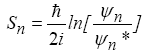(24)

The QIETD (23) can be written as a function of the wave function as following: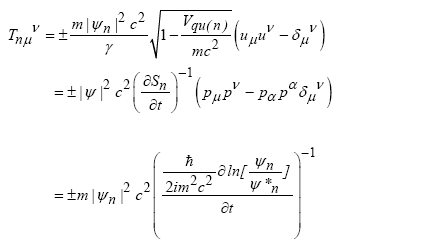(25)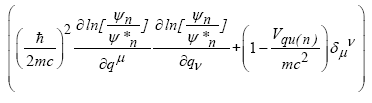Charged field

In the case of a charged boson field, equations (1-3) read, respectively,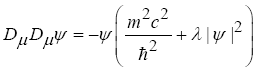(26)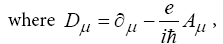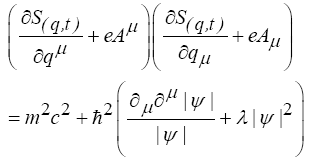(27)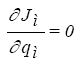(28)

where the 4-current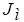reads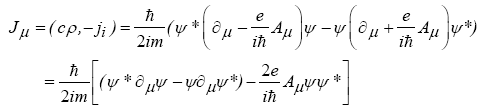(29)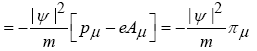and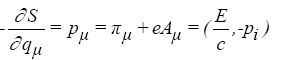(30)

(where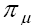is the mechanical momentum) [11,13] and where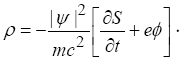(31)

Moreover, analogously to (9,17-19), from (27) it follows that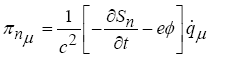(32)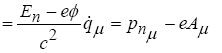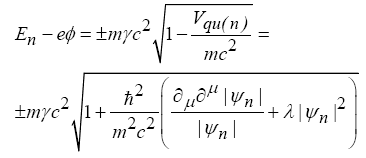(33)

to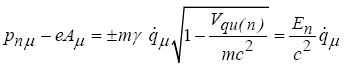(34)

and to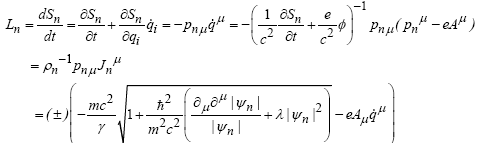(35)

that, by using (24), as a function of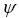and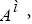reads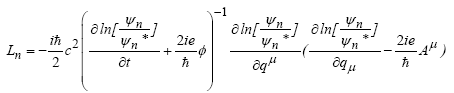Moreover, with the help of (24,29,32-34) it follows that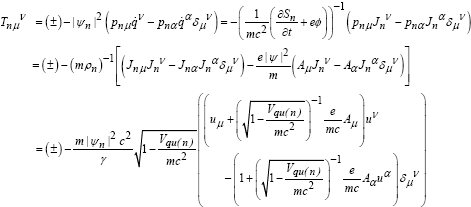(36)

that, by using (24,29,34) we can express as a function of the wave function as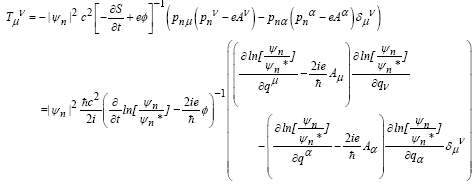(37)

The above equations are coupled to the Maxwell one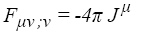(38)

(where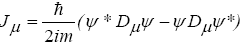is the current of the charged particles) where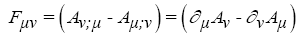(39)

and where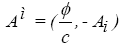(40)

is the potential 4-vector,

#### Non-Euclidean Generalization

The quartic self-interaction is introduced in the KGE in order to describe the states of charged (±1) bosons (e.g., mesons) . The importance of having the hydrodynamic description of bosons  lies in the fact that it allows to derive its quantum energy-impulse tensor that can couple them to the Einstein quantum-gravitational equation .

The generalization of the quantum hydrodynamic formalism to the non-Euclidean space-time can be obtained by using the General Physics Covariance postulate [11,16]. By using it, it is possible to derive the non-Euclidean expression of the hydrodynamic model of the KGE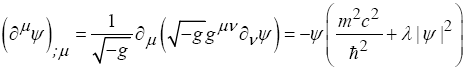(41)

Equations (2-3) in a non- Euclidean space read, respectively,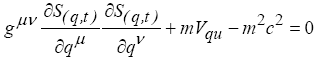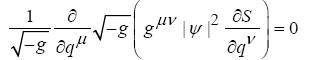(42)

where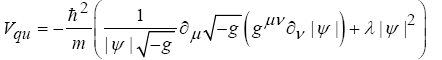(43)

Moreover, by using the definition of the Lagrangean function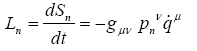(44)

the covariant form of the motion equations (14-15) reads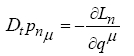(45)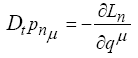(46)

where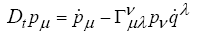(47)

is the total covariant derivative respect the time and where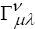are the Christoffel symbols.

Equations (45-46) leads to the motion equation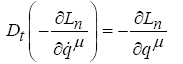(48)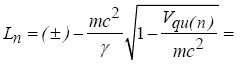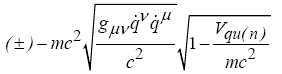(49)

From (48) it follows that the motion equation reads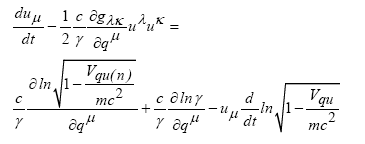(50)

where the stationary condition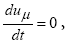that determines the balance between the “force” of gravity and that one of the quantum potential, leads to the stationary equation for the Eigen states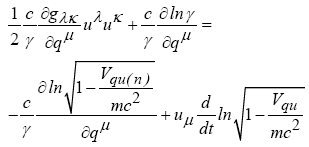(51)

where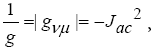where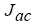is Jacobean of the transformation of the Galilean co-ordinates to non-Euclidean ones and where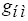is the metric tensor defined by the quantum gravitational equation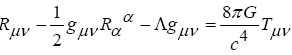(52)

where the quantum energy impulse tensor density reads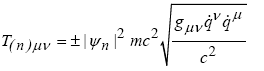(53)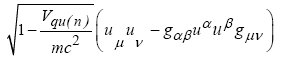and where the cosmological energy-impulse density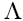, for Eigen states, reads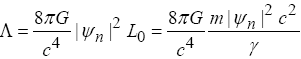(54)

where, for scalar uncharged particles leads to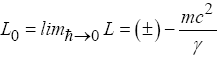(55)

Finally, it is worth noting that, as a function of the quantum field, the quantum energy impulse tensor density reads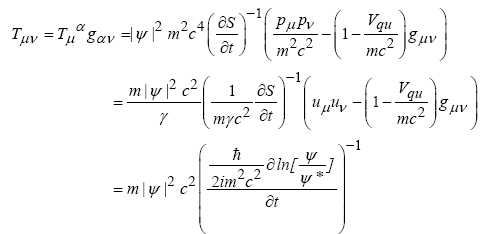(56)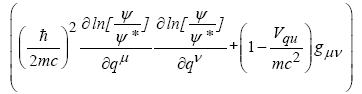Charged boson in non-Euclidean space-time

The KGE in non-Euclidean space-time for electromagnetic charged boson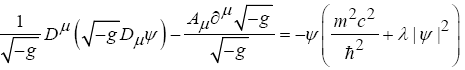(57)

leads to the hydrodynamic system of equations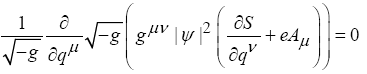(58)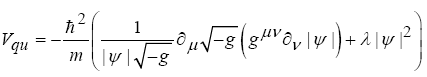(59)

where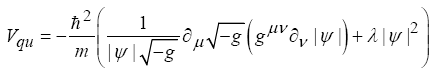(60)

Moreover, the Lagrangean motion equations read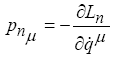(61)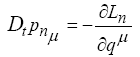(62)

where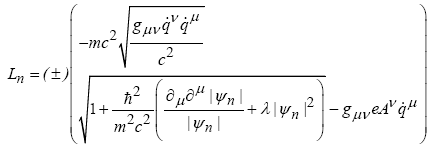(63)

and to the QIETD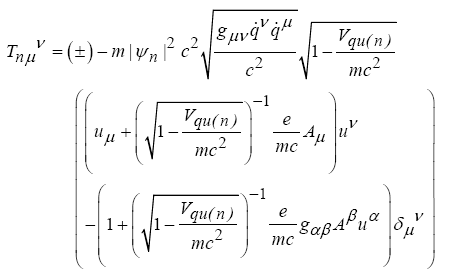(64)

#### Conclusion

The hydrodynamic approach allows obtaining the quantum energy-impulse tensor density as a function the field of the particle.

The biunique correspondence between the standard quantum mechanics and the hydrodynamic representation [1-6,17] warrants that the quantum energy-impulse tensor density can be independently defined by the used formalism.

In this work the quantum energy-impulse tensor, for massive bosons described by a KGE with self-interacting field is derived for defining the coupling with the quantum gravitational equation.

#### References

Citation: Chiarelli P (2017) The Hydrodynamic Representation of the Klein-Gordon Equation with Self-Interacting Field. J Astrophys Aerospace Technol 5: 148. Doi: 10.4172/2329-6542.1000148

Copyright: © 2017 Chiarelli P. This is an open-access article distributed under the terms of the Creative Commons Attribution License, which permits unrestricteduse, distribution, and reproduction in any medium, provided the original author and source are credited.

Select your language of interest to view the total content in your interested language

##### Recommended Journals
Viewmore
###### Article Usage
• Total views: 1329
• [From(publication date): 0-2017 - Nov 14, 2019]
• Breakdown by view type
• HTML page views: 1254Can't read the image? click here to refresh